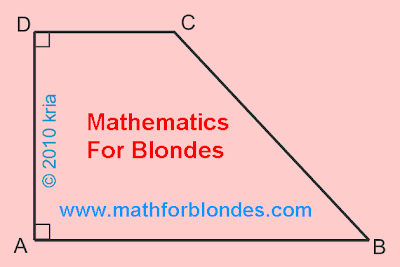## Pages

Showing posts with label trapezoid. Show all posts
Showing posts with label trapezoid. Show all posts

## 2.10.2018

### Trapezoid

In Wikipedia there is a page of a trapezoid. In the drawing it is shown that any trapezoid can be turned into a rectangle.Trapezoid
Let's look at algebra. These are formulas for calculation of lengths of diagonals of a trapezoid.Diagonals of trapezoid
We substitute in these formulas data for a rectangle.Diagonals of rectangle
If to trust these formulas, the rectangle has no diagonals. Even schoolboys or schoolgirls can make what mathematicians couldn't make – to execute verification of the solution of a task. It is the actual level of modern mathematics – any statement of mathematicians can be false.

Height of a trapezoid

Height of a trapezoid is determined by the area of a triangle. The area of a triangle is calculated on Heron's formula. The sizes of the parties of a trapezoid allow to receive a triangle which has the same height as a trapezjid. The cunning trick of mathematicians allows to calculate length of diagonals of a trapezoid.Height of a trapezoid
When the legs of a trapezoid are parallel, the triangle disappears and the cunning trick ceases to work. If to determine height of a trapezoid by the area of a trapezoid, then no problems arise upon transition to a rectangle or a parallelogram.Height of a rectangle
Conclusion: cunning tricks of mathematicians can result in false results.

## 8.14.2010

### How does a rectangular trapezoid look?

A rectangular trapezoid has such kind:It is a rectangular trapeze.
At a rectangular trapezoid I always am two lines of corner. On a picture direct corners are marked little squares in tops A and D. Direct corners can be situated in two any contiguous tops of trapezoid.It is a rectangular trapeze.
On this picture of rectangular trapezoid direct corners are located in tops B and С.

In mathematics there is determination of rectangular trapezoid, which over is brought and in Wikipedia: the rectangular is name a trapezoid at which even one corner of line. This classic determination of rectangular trapezoid, bluntly rewritten from sacred mathematical books. In Euclidean geometry a rectangular trapezoid always has two lines of corner, as two sides trapezoids, called grounds, are parallel. Segment BC, formative a direct corner with founding of АВ, will be always perpendicular to the second founding of CD. With one or three direct corners there can be only a curvilinear rectangular trapezoid, and it is quite another other class of geometrical figures already.

For blondes helped to draw kria, author of blog kria-tiv.